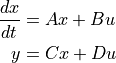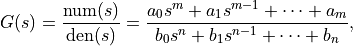# Library conventions¶

The python-control library uses a set of standard conventions for the way that different types of standard information used by the library. Throughout this manual, we assume the control package has been imported as ct.

## LTI system representation¶

Linear time invariant (LTI) systems are represented in python-control in state space, transfer function, or frequency response data (FRD) form. Most functions in the toolbox will operate on any of these data types and functions for converting between compatible types is provided.

### State space systems¶

The StateSpace class is used to represent state-space realizations of linear time-invariant (LTI) systems:where u is the input, y is the output, and x is the state.

To create a state space system, use the ss() function:

sys = ct.ss(A, B, C, D)


State space systems can be manipulated using standard arithmetic operations as well as the feedback(), parallel(), and series() function. A full list of functions can be found in Function reference.

### Transfer functions¶

The TransferFunction class is used to represent input/output transfer functionswhere n is generally greater than or equal to m (for a proper transfer function).

To create a transfer function, use the tf() function:

sys = ct.tf(num, den)


Transfer functions can be manipulated using standard arithmetic operations as well as the feedback(), parallel(), and series() function. A full list of functions can be found in Function reference.

### FRD (frequency response data) systems¶

The FrequencyResponseData (FRD) class is used to represent systems in frequency response data form.

The main data members are omega and fresp, where omega is a 1D array with the frequency points of the response, and fresp is a 3D array, with the first dimension corresponding to the output index of the FRD, the second dimension corresponding to the input index, and the 3rd dimension corresponding to the frequency points in omega.

FRD systems have a somewhat more limited set of functions that are available, although all of the standard algebraic manipulations can be performed.

The FRD class is also used as the return type for the frequency_response() function (and the equivalent method for the StateSpace and TransferFunction classes). This object can be assigned to a tuple using:

mag, phase, omega = response


where mag is the magnitude (absolute value, not dB or log10) of the system frequency response, phase is the wrapped phase in radians of the system frequency response, and omega is the (sorted) frequencies at which the response was evaluated. If the system is SISO and the squeeze argument to frequency_response() is not True, magnitude and phase are 1D, indexed by frequency. If the system is not SISO or squeeze is False, the array is 3D, indexed by the output, input, and frequency. If squeeze is True then single-dimensional axes are removed. The processing of the squeeze keyword can be changed by calling the response function with a new argument:

mag, phase, omega = response(squeeze=False)


### Discrete time systems¶

A discrete time system is created by specifying a nonzero ‘timebase’, dt. The timebase argument can be given when a system is constructed:

• dt = 0: continuous time system (default)

• dt > 0: discrete time system with sampling period ‘dt’

• dt = True: discrete time with unspecified sampling period

• dt = None: no timebase specified

Only the StateSpace, TransferFunction, and InputOutputSystem classes allow explicit representation of discrete time systems.

Systems must have compatible timebases in order to be combined. A discrete time system with unspecified sampling time (dt = True) can be combined with a system having a specified sampling time; the result will be a discrete time system with the sample time of the latter system. Similarly, a system with timebase None can be combined with a system having a specified timebase; the result will have the timebase of the latter system. For continuous time systems, the sample_system() function or the StateSpace.sample() and TransferFunction.sample() methods can be used to create a discrete time system from a continuous time system. See Utility functions and conversions. The default value of dt can be changed by changing the value of control.config.defaults[‘control.default_dt’].

### Conversion between representations¶

LTI systems can be converted between representations either by calling the constructor for the desired data type using the original system as the sole argument or using the explicit conversion functions ss2tf() and tf2ss().

## Simulating LTI systems¶

A number of functions are available for computing the output (and state) response of an LTI systems:

 initial_response(sys[, T, X0, input, ...]) Compute the initial condition response for a linear system. step_response(sys[, T, X0, input, output, ...]) Compute the step response for a linear system. impulse_response(sys[, T, X0, input, ...]) Compute the impulse response for a linear system. forced_response(sys[, T, U, X0, transpose, ...]) Compute the output of a linear system given the input.

Each of these functions returns a TimeResponseData object that contains the data for the time response (described in more detail in the next section).

The forced_response() system is the most general and allows by the zero initial state response to be simulated as well as the response from a non-zero intial condition.

In addition the input_output_response() function, which handles simulation of nonlinear systems and interconnected systems, can be used. For an LTI system, results are generally more accurate using the LTI simulation functions above. The input_output_response() function is described in more detail in the Input/output systems section.

### Time series data¶

A variety of functions in the library return time series data: sequences of values that change over time. A common set of conventions is used for returning such data: columns represent different points in time, rows are different components (e.g., inputs, outputs or states). For return arguments, an array of times is given as the first returned argument, followed by one or more arrays of variable values. This convention is used throughout the library, for example in the functions forced_response(), step_response(), impulse_response(), and initial_response().

Note

The convention used by python-control is different from the convention used in the scipy.signal library. In Scipy’s convention the meaning of rows and columns is interchanged. Thus, all 2D values must be transposed when they are used with functions from scipy.signal.

The time vector is a 1D array with shape (n, ):

T = [t1,     t2,     t3,     ..., tn    ]


Input, state, and output all follow the same convention. Columns are different points in time, rows are different components:

U = [[u1(t1), u1(t2), u1(t3), ..., u1(tn)]
[u2(t1), u2(t2), u2(t3), ..., u2(tn)]
...
...
[ui(t1), ui(t2), ui(t3), ..., ui(tn)]]


(and similarly for X, Y). So, U[:, 2] is the system’s input at the third point in time; and U or U[1, :] is the sequence of values for the system’s second input.

When there is only one row, a 1D object is accepted or returned, which adds convenience for SISO systems:

The initial conditions are either 1D, or 2D with shape (j, 1):

X0 = [[x1]
[x2]
...
...
[xj]]


Functions that return time responses (e.g., forced_response(), impulse_response(), input_output_response(), initial_response(), and step_response()) return a TimeResponseData object that contains the data for the time response. These data can be accessed via the time, outputs, states and inputs properties:

sys = ct.rss(4, 1, 1)
response = ct.step_response(sys)
plot(response.time, response.outputs)


The dimensions of the response properties depend on the function being called and whether the system is SISO or MIMO. In addition, some time response function can return multiple “traces” (input/output pairs), such as the step_response() function applied to a MIMO system, which will compute the step response for each input/output pair. See TimeResponseData for more details.

The time response functions can also be assigned to a tuple, which extracts the time and output (and optionally the state, if the return_x keyword is used). This allows simple commands for plotting:

t, y = ct.step_response(sys)
plot(t, y)


The output of a MIMO LTI system can be plotted like this:

t, y = ct.forced_response(sys, t, u)
plot(t, y, label='y_0')
plot(t, y, label='y_1')


The convention also works well with the state space form of linear systems. If D is the feedthrough matrix (2D array) of a linear system, and U is its input (array), then the feedthrough part of the system’s response, can be computed like this:

ft = D @ U


Finally, the to_pandas() function can be used to create a pandas dataframe:

df = response.to_pandas()


The column labels for the data frame are time and the labels for the input, output, and state signals (u[i], y[i], and x[i] by default, but these can be changed using the inputs, outputs, and states keywords when constructing the system, as described in ss(), tf(), and other system creation function. Note that when exporting to pandas, “rows” in the data frame correspond to time and “cols” (DataSeries) correspond to signals.

## Package configuration parameters¶

The python-control library can be customized to allow for different default values for selected parameters. This includes the ability to set the style for various types of plots and establishing the underlying representation for state space matrices.

To set the default value of a configuration variable, set the appropriate element of the control.config.defaults dictionary:

ct.config.defaults['module.parameter'] = value


The ~control.config.set_defaults function can also be used to set multiple configuration parameters at the same time:

ct.config.set_defaults('module', param1=val1, param2=val2, ...]


Finally, there are also functions available set collections of variables based on standard configurations.

Selected variables that can be configured, along with their default values:

• freqplot.dB (False): Bode plot magnitude plotted in dB (otherwise powers of 10)

• freqplot.deg (True): Bode plot phase plotted in degrees (otherwise radians)

• freqplot.Hz (False): Bode plot frequency plotted in Hertz (otherwise rad/sec)

• freqplot.grid (True): Include grids for magnitude and phase plots

• freqplot.number_of_samples (1000): Number of frequency points in Bode plots

• freqplot.feature_periphery_decade (1.0): How many decades to include in the frequency range on both sides of features (poles, zeros).

• statesp.use_numpy_matrix (True): set the return type for state space matrices to numpy.matrix (verus numpy.ndarray)

• statesp.default_dt and xferfcn.default_dt (None): set the default value of dt when constructing new LTI systems

• statesp.remove_useless_states (True): remove states that have no effect on the input-output dynamics of the system

Additional parameter variables are documented in individual functions

Functions that can be used to set standard configurations:

 Reset configuration values to their default (initial) values. Use Feedback Systems (FBS) compatible settings. Use MATLAB compatible configuration settings. use_numpy_matrix([flag, warn]) Turn on/off use of Numpy matrix class for state space operations. use_legacy_defaults(version) Sets the defaults to whatever they were in a given release.